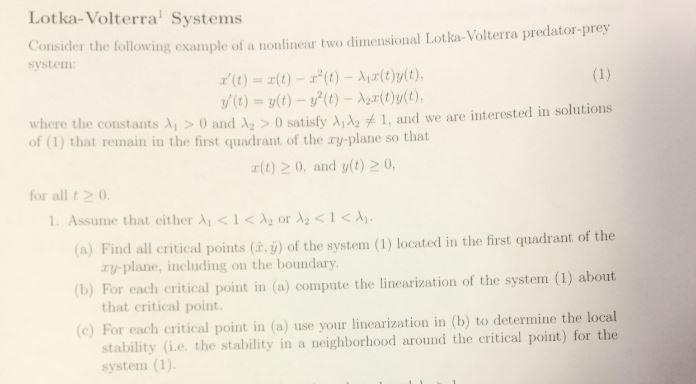# Lotka-Volterra SystemsConsider the following example of a nonlinear two dimensional Lotka- Volterra predator-preysystemr(t) =エ(t)-r'(t)-Air(t)y(t),where the constants λί > 0 and Az > 0 satisfy λιλ2 and we are interested in solutionsof (1) that remain in the first quadrant of the ry-plane so thatr(t) 20, and yt) 20,for all t01. Assume that either λί < 1CA2 or λ2 < 1

Question
123 views

Pls explain to me step by step. pls dont skip any steps. thankshelp_outlineImage TranscriptioncloseLotka-Volterra Systems Consider the following example of a nonlinear two dimensional Lotka- Volterra predator-prey system r(t) =エ(t)-r'(t)-Air(t)y(t), where the constants λί > 0 and Az > 0 satisfy λιλ2 and we are interested in solutions of (1) that remain in the first quadrant of the ry-plane so that r(t) 20, and yt) 20, for all t0 1. Assume that either λί < 1CA2 or λ2 < 1<λι. (a) Find all critical points (à. ) of the system (1) located in the first quadrant of the (b) For each critical point in (o) compute the linearization of the system (1) about (e) For each critical point in (a) use your linearization in (b) to determine the local ry-plane, including on the boundary that critical point. stability (i.e. the stability in a neighborhood around the critical point) for the system (1) fullscreen
check_circle

Step 1

To study the critical points of the given system

Step 2
Step 3

### Want to see the full answer?

See Solution

#### Want to see this answer and more?

Solutions are written by subject experts who are available 24/7. Questions are typically answered within 1 hour.*

See Solution
*Response times may vary by subject and question.
Tagged in

### Math